# AP Board 7th Class Maths Solutions Chapter 10 Construction of Triangles Ex 10.2

SCERT AP 7th Class Maths Solutions Pdf Chapter 10 Construction of Triangles Ex 10.2 Textbook Exercise Questions and Answers.

## AP State Syllabus 7th Class Maths Solutions 10th Lesson Construction of Triangles Ex 10.2

Question 1.
Construct ∆ABC with measurements AB = 4.5 cm, BC = 6 cm and ∠B=75°
Given measurements of ∆ABC are AB = 4.5 cm, BC = 6 cm and ∠B = 75°.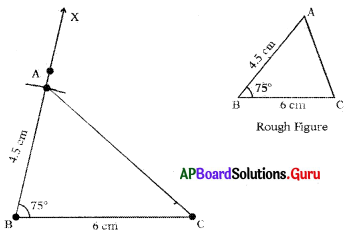Steps of Construction :

1. Draw a rough sketch of triangle and label it with given measurements.
2. Draw a line segment with BC = 6 cm.
3. Draw a ray $$\overrightarrow{\mathrm{BX}}$$ such that ∠CBX = 15°.
4. Draw an arc with centre B and radius 4.5 cm, to intersect $$\overrightarrow{\mathrm{BX}}$$ at point A.
Thus, required ∆ABC is constructed with the given measurements.Question 2.
Construct an isosceles triangle with measurements DE = 7 cm, EF = 7 cm and ∠E = 60°
Given measurements of ∆DEF are DE = 7 cm, EF = 7 cm and ∠E = 60°.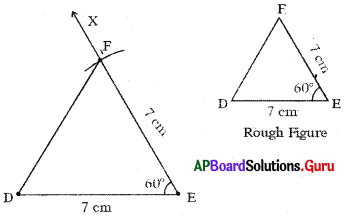Steps of Construction:

1. Draw a rough sketch of triangle and label it with given measurements.
2. Draw a line segment with DE = 7 cm.
3. Draw a ray $$\overrightarrow{\mathrm{EX}}$$ such that ∠DEX = 60°.
4. Draw an arc with centre E and radius 7 cm to intersect $$\overrightarrow{\mathrm{EX}}$$ at point F.
5. Join DF.
Hence, required ∆DEF is constructed with the given measurements.

Question 3.
Draw a triangle with,measurements ∠B = 50°, AB = 3 cm and AC = 4 cm.
Given measurements of a triangle are ∠B = 50°, AB = 3 cm and AC = 4cm..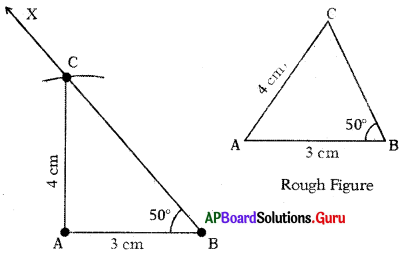Steps of Construction:

1. Draw a rough sketch of triangle and label it with given measurements.
2. Draw a line segment with AB = 3 cm.
3. Draw a ray $$\overrightarrow{\mathrm{BX}}$$ such that ∠ABX = 50°.
4. Draw an arc with centre A and radius 4 cm to intersect $$\overrightarrow{\mathrm{BX}}$$ at point C.
5. Join AC.
Hence, required ∆ABC is constructed with the given measurements.

Question 4.
Construct a right angled triangle in which, XY = 5 cm, XZ = 6 cm and right tingle at X.
Given measurements of ∆XYZ are XY = 5 cm, XZ = 6 cm and ZX = 90° (∠YXZ = 90°)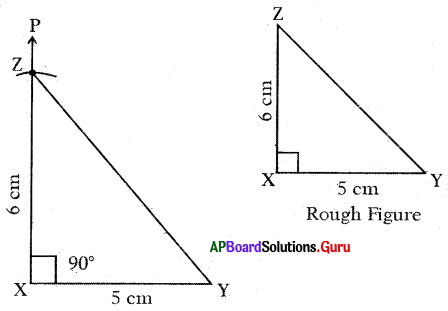Steps of Construction:

1. Draw a rough sketch of triangle arid label it with given measurements.
2. Draw a line segment with XY = 5 cm.
3. Draw a ray $$\overrightarrow{\mathrm{XP}}$$ such that ∠YXP = 90°.
4. Draw an arc with centre X and radius 6 cm to intersect XP at point Z.
5. Join YZ.
Hence, required triangle ∆XYZ is constructed with the given measurements.Question 5.
Construct a right angled with measurements ABC, ∠B = 90°, AB = 8 cm and AC = 10 cm.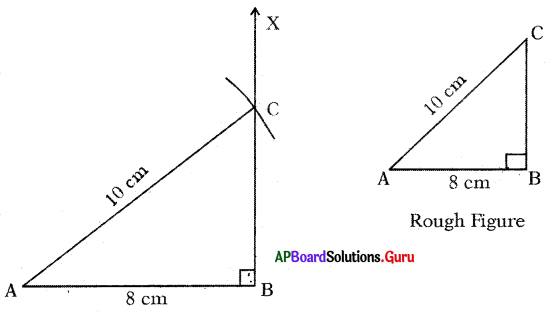3. Draw a ray $$\overrightarrow{\mathrm{BX}}$$ such that ∠ABX = 90°.
4. Draw an arc with centre A and radius 10 cm to intersect $$\overrightarrow{\mathrm{BX}}$$ at point C.